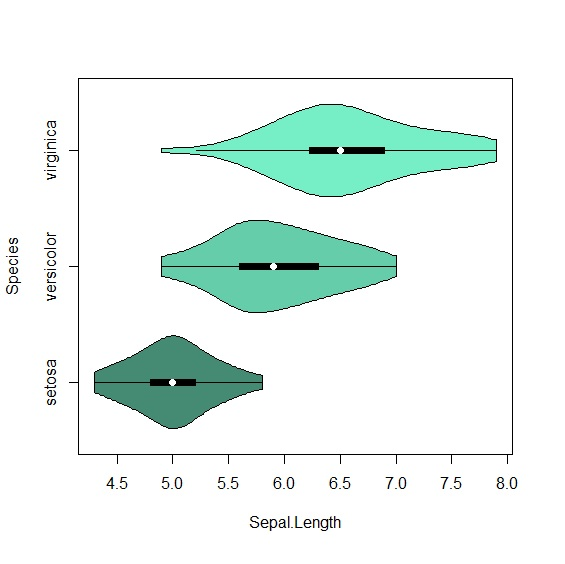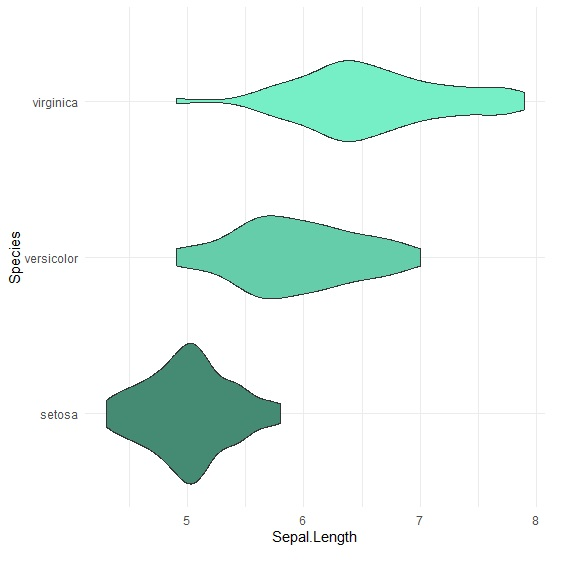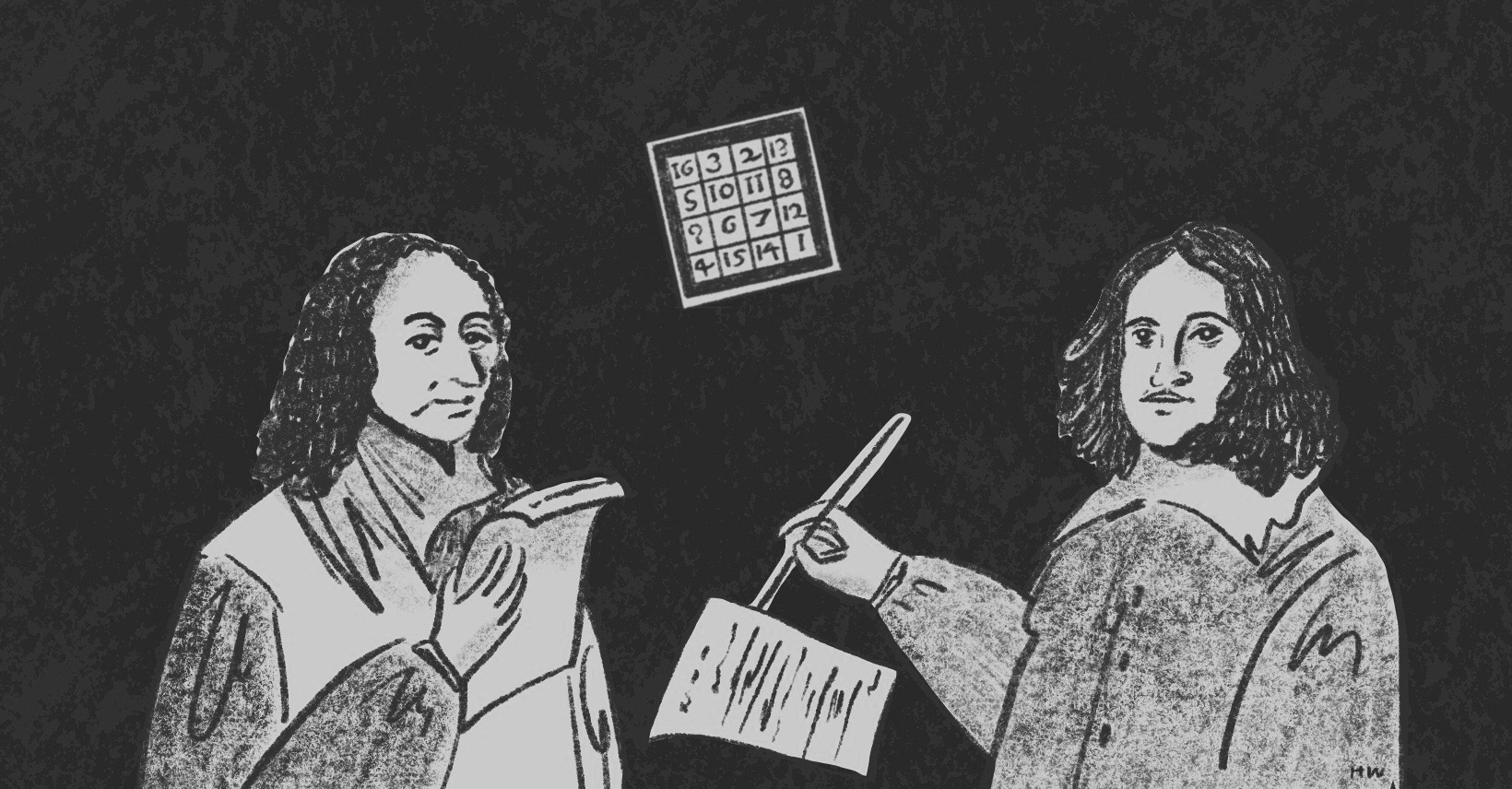×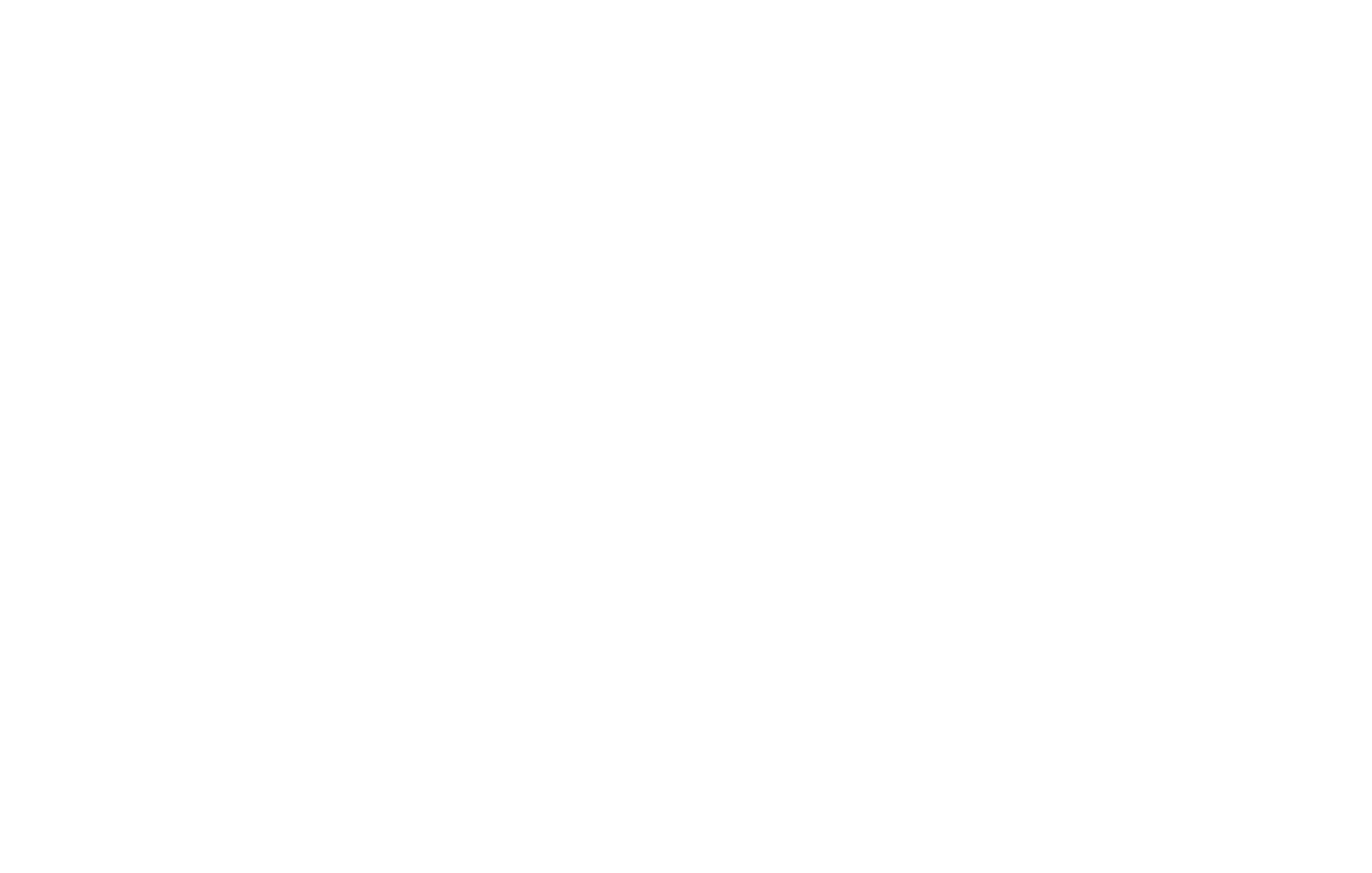資料管理 統計分析 相關資源 巨人肩膀 語法索引 關於作者

## 簡介

• boxplot() -R Base箱型圖
• geom_boxplot() -ggplot2箱型圖
• geom_jitter -ggplot2資料點
• vioplot() -R Base小提琴圖
• geom_violin() -ggplot2小提琴圖

## 箱型圖

boxplot()是R Base繪製箱型圖的指令。這裡一樣以鳶尾花資料檔示範如何在R畫出箱型圖。

 > data(iris) > attach(iris) > boxplot(Sepal.Length~Species, col=c("aquamarine4","aquamarine3","aquamarine2")) 

 > p<-ggplot(iris, aes(x=Species, y=Sepal.Length, fill=Species))+ + geom_boxplot()+ + scale_fill_manual(values=c("aquamarine4","aquamarine3","aquamarine2"))+ + theme_minimal()+ + theme(legend.position="none") > p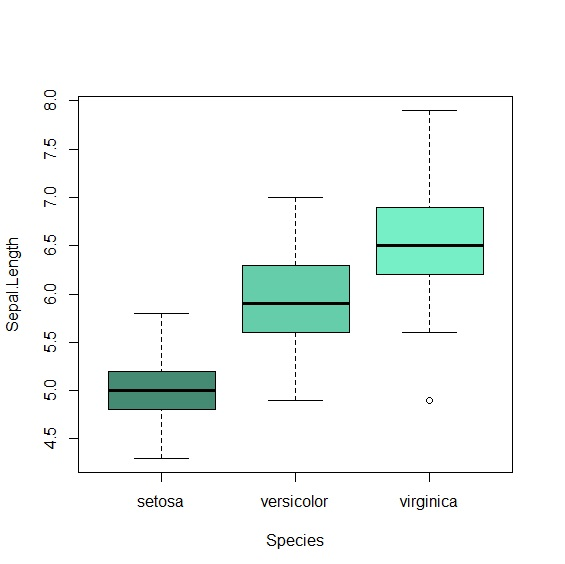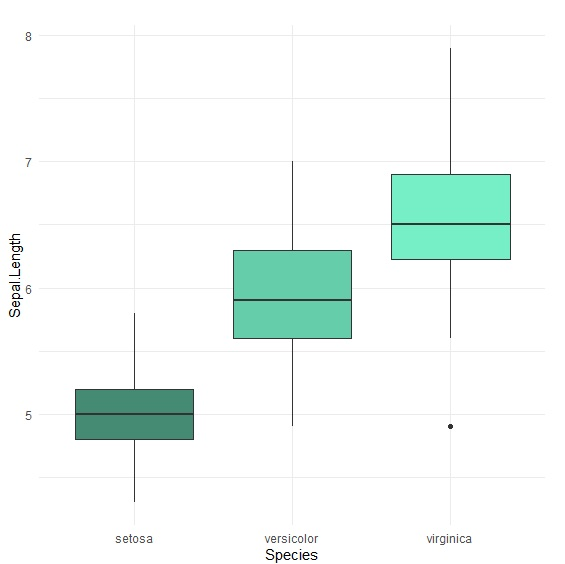## 資料箱型圖

ggplot2有個不錯的功能，可以替箱型圖加上真實資料分布位置，讓我們直接用肉眼看到資料分布，避免箱型圖隱藏資料訊息。要使用這個功能也非常簡單，只要在原本的箱型圖上加上geom_jitter()圖層就可以了。geom_jitter()其實就是geom_point(position="jitter")的快捷方法。

 > p<-ggplot(iris, aes(x=Species, y=Sepal.Length, fill=Species))+ + geom_boxplot()+ + scale_fill_manual(values=c("aquamarine4","aquamarine3","aquamarine2"))+ + theme(legend.position="none")+ + geom_jitter(color="black", size=0.5, alpha=0.8)+ + theme_minimal() > p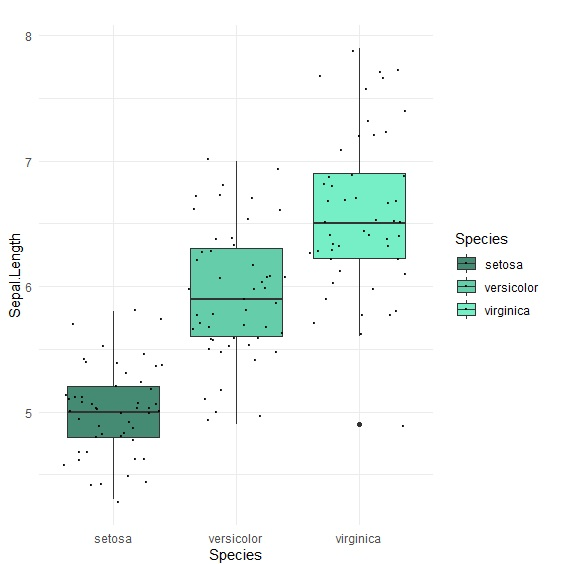## 群組箱型圖

 > attach(ToothGrowth) > boxplot(len~supp*dose, col=c("aquamarine4","aquamarine3"))+ 

ggplot2群組箱型圖，其實只要將第二個組別設定在fill=variable填色裡即可。

 > p<-ggplot(ToothGrowth, aes(x=as.factor(dose), y=len, fill=supp))+ + geom_boxplot()+ + scale_fill_manual(values=c("aquamarine4","aquamarine3"))+ + theme_minimal() > p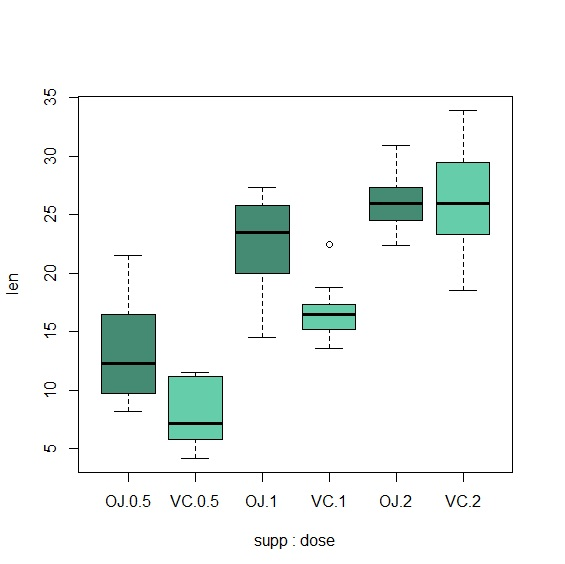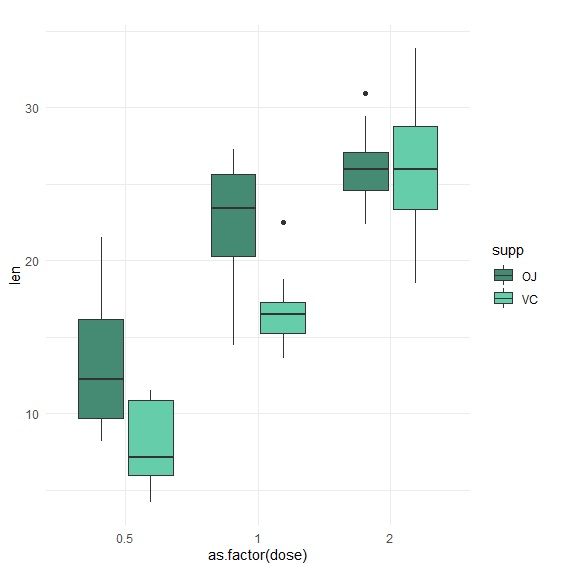## 小提琴圖

 > library(vioplot) > vioplot(Sepal.Length~Species, data=iris, horizontal=T, col=c("aquamarine4","aquamarine3","aquamarine2")) 

 > p<-ggplot(iris, aes(x=Species, y=Sepal.Length, fill=Species))+ + geom_violin()+ + scale_fill_manual(values=c("aquamarine4","aquamarine3","aquamarine2"))+ + coord_flip()+ + theme_minimal()+ + theme(legend.position="none") > p